Statistical Concepts and Market Return | IFT World
IFT Notes for Level I CFA® Program
IFT Notes for Level I CFA® Program

# Part 3

## 7. Measures of Dispersion

In this segment we look at measures that tell us how spread out or dispersed our data might be.

### 7.1 The Range

The range is the difference between the maximum and minimum values in a data set. It is expressed as:

Range = Max value – Min Value

If the annual returns data is: 10%, -5%, 10%, 25%. What is the range?

Here the maximum return is 25% and the minimum return is -5%. The range is 25% – (-5%) = 30%.

### 7.2 The Mean Absolute Deviation

It is the average of the absolute values of deviations from the mean. It is expressed as: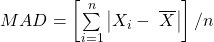where: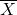is the sample mean and n is the number of observations in the sample.

Example

Consider the following data set: 8, 12, 10, 8 and 5. Calculate the mean absolute deviation.

Solution:= (8 + 12 + 10 + 8 + 5) / 5 = 8.6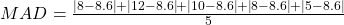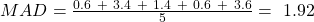### 7.3 Population Variance and Population Standard Deviation

Population variance is the mean of the squared deviations from the mean. The population variance is computed using all members of a population. It is expressed as: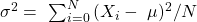where: µ is the population mean and N is the size of the population

Population standard deviation is defined as the positive square root of the population variance.

Example

Calculate the population variance and standard deviation for this dataset: 2%, 5%, 4%, 7%, 8%, 8%, 12%, 10%, 8%, and 5%.

Solution: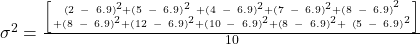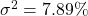Population standard deviation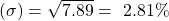### 7.4 Sample Variance and Sample Standard Deviation

Sample variance applies when we are dealing with a subset, or sample, of the total population. It is expressed as: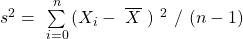where: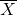is the sample mean and n is the number of observations  in the sample.

Sample standard deviation is defined as the positive square root of the sample variance.

Example

Calculate the sample variance for the following data set: 8, 12, 10, 8 and 5.

Solution: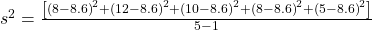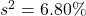The sample standard deviation is the positive square root of the sample variance.  For the sample data given above,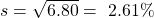Using a financial calculator to calculate variance and standard deviations

The population and sample standard deviation can easily be computed using a financial calculator. Assume the following data set: 10%, -5%, 10%, 25%, the calculator key strokes are shown below:

 Keystrokes Description Display [2nd] [DATA] Enters data entry mode [2nd] [CLR WRK] Clears data register X01 10 [ENTER] X01 = 10 [↓] [↓] 5+/- [ENTER] X02 = -5 [↓] [↓] 10 [ENTER] X03 = 10 [↓] [↓] 25 [ENTER] X04 = 25 [2nd] [STAT] [ENTER] Puts calculator into stats mode [2nd] [SET] Press repeatedly till you see à 1-V [↓] Number of data points N = 4 [↓] Mean X = 10 [↓] Sample standard deviation Sx = 12.25 [↓] Population standard deviation σx = 10.61

Notice that the calculator gives both the sample and the population standard deviation.  On the exam we will have to determine whether we are dealing with population or sample data and choose the appropriate value.

### 7.5 Semivariance, Semideviation, and Related Concepts

Instructor’s Note: Semivariance and semideviation are not emphasized in the learning outcomes and have a very low probability of being tested on the Level I exam. Nevertheless, a brief explanation is given below.

Variance and standard deviation of returns take account of returns above and below the mean, but often investors are concerned only with downside risk, for example returns below the mean. As a result, analysts have developed semivariance, semideviation and related dispersion measures that focus on downside risk. Semivariance is defined as the average squared deviation below the mean. Semideviation is the positive square root of semivariance.

### 7.6 Chebyshev’s Inequality

According to Chebyshev’s inequality, the proportion of the observations within k standard deviations of the arithmetic mean is at least: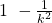for all k > 1.

To find out what percent of the observations must be within two standard deviations of the mean we simply plug into the formula and get: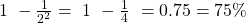.  Hence, at least 75% of the data will be between two standard deviations of the mean.

Chebyshev’s inequality can be used to measure maximum amount of dispersion, regardless of the shape of the distribution. Notice that here we do not make any assumptions about whether the distribution is normal or not normal. This inequality applies across all distributions.

### 7.7 Coefficient of Variation

Coefficient of variation expresses how much dispersion exists relative to the mean of a distribution and allows for direct comparison of dispersion across different data sets. It is used in investment analysis to compare relative risks. When evaluating investments, a lower value is better. Coefficient of variation is expressed as: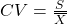where: s = sample standard deviation of a set of observations and= sample mean

Example

Investment A has a mean return of 7% and a standard deviation of 5%. Investment B has a mean return of 12% and a standard deviation of 7%. Calculate the coefficients of variation.

Solution

The coefficients of variation can be calculated as follows: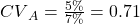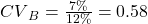This metric shows that Investment A is riskier than Investment B.

LIVE WEEKEND CLASSES *For 2024 Exam candidates*
This is default text for notification bar